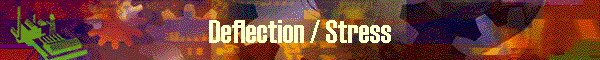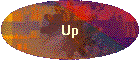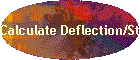### Philosophy of calculation

This function calculates rod deflection and stress according to the following understanding and assumptions.

### For the rod which is cast (Fly Rod and Lure Rod)

All the rod parts including rod blank assume to receive two kind of accelerations, here the rod is hold vertically translated aside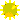gravity acceleration toward vertical directionhorizontal acceleration specified in G equivalent unit

Forth of Inertia

Forth of inertia is the force which is produced by inertia when the material is moved.  Inertia is the physical nature which tries to remain to stay at original location or to remain moving. when it is in the motion.

Forth of inertia is calculated in the formula of F=ma, where F is force, m is mass and "a" is the acceleration of movement.

The weight on the earth is calculated as follows;  W is the unit of weight which we are using every day in gram, kilo gram , ounce or pound.

W=mg  where m is mass, "g" is gravity acceleration (caused by pulling force of earth).

Let's see examples.  We assume the black square in the picture below as a train.  In the cart of train, a weight is hung down from the ceiling of the cart, and a rod stands vertically with its end is fixed to the floor of the cart.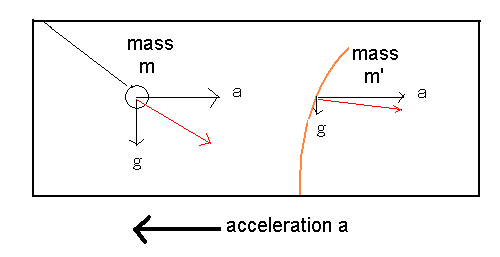Here we assume that the mass of the weight =m, the mass of a part of rod section (e.g. 1" section)=m'.   We assume a rod is subdivided into 1" sections.

Now, the train suddenly moved toward the direction which a thick arrow shows in the picture.  As a result of the train's movement, acceleration= a was given to the train.

Inertia works on everything which is connected to the train cart.  The connected part of thing in the cart moves together with the cart though it is receiving the force of inertia.  Since the force of train to move forward is larger enough than the forth of inertia, the connected part of the rod and the weight have to move.

Thus the weight and the rod receives the same amount of acceleration=-a (towards opposite direction to the train) because of the nature of inertia=a.

The opposite acceleration of "-a" generate the force of inertia to the weight and the rod as, F=-ma and F=-m'a respectively.

On the other hand, the weight and the rod receives the force of gravity vertically, the force is calculated as follows;  W=mg and W=m'g, these are the weight on the earth.

The weight hung from ceiling, receives two directions of forces by "-ma" horizontally and "mg" vertically, and balances at the red line angle where it is considered that the force "-ma" (vector of "-a") and force "mg" (vector of "g")  are added together and produced the added force and direction. ( of red line).

The same thing can be seen on the rod.   The horizontal force "-m'a" and vertical force "m'g" are added as vector, and produces the added force and direction (of red line).

This added force shown as a red line will pull the rod toward the red line and generate moment at each point of the butt side, though the red line direction must be translated to the force which is perpendicular to the rod angle.

The reason why the rod does not shape like the straight line connected to the weight, is because the rod material has rigidity.  The rigidity resists to the force of inertia and the each of rod sections balances at a certain angle where moment becomes rigidity, respectively.

Dynamic calculation continues this kind of calculations to adjust the force angles to be perpendicular toward the deflected rod angle from time to time as well as calculating the magnitude of added force of (m'g + -m'a).  Here - (minus) only means the direction of force not the number is minus.  The dynamic calculation means the iteration of this kind of adjustment of angles from time to time, not to calculate only once.
Moment=>Deflection=>Moment=>Deflection until the rod is balanced.

In the actual casting, we have to consider the force generated by the flow of air or wind.  The air resistance is proportioned with the area facing to the air, DynaRod is factoring it by the size of dimension.  Lvalue in Bamboo Attribute table includes the factor.

### For the rod which is hold horizontally (Boat Rod)

The following picture shows that the rod is held horizontally and not moved up and down.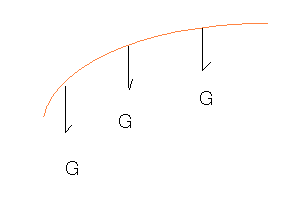The horizontally held static rod receives the same gravity acceleration always at all the part of the rod.  DynaRod defines the unit of acceleration as 1G=g where g is gravity acceleration, that is 9.8m/power of second.  For the acceleration toward horizontal direction, it can be translated in G unit by G= a/g since acceleration does not have such constant.  In outer space, there is no difference between "g" and "a", we need the same unit of measure for the acceleration towards any direction.

So again, we defines, G=a/g

For static calculation for a static rod model which does not move, we may just need to consider only "g", as W=mg.

However, to answer to the desire that we want to know how a boat rod deflects when the boat rod is moved upward when a fish is on, or invite fishes by the move, DynaRod dare not to consider that the rod receives "g", but receives an artificial 1G instead of natural "g".  Thus the horizontal rod model can be moved upward in any speed which receives corresponding G numbers according to the speed.  G greater than 1 means that the rod model becomes a dynamic model.

So, for a boat rod, DynaRod does not assume both of "a" and "g" but assumes only "g" though it is artificial 1G.

### The meaning to multiply the weight of rod parts by 4

Let's think about the meaning what Everett Garrison did on his model by multiplying 4 to the weight of rod parts.  Someone insists that this is a hypothetical model.  Is it true?

Let's think.

Now we assume a rod which is held horizontally.  The rod is not moved by a human hand.

The entire rod including blank and rod parts is receiving gravity acceleration of "g".

Now for every part we can weigh its weight on the earth as W=mg.  W can be measured by a scale.  As g=9.8m/power of second in metric, we can calculate "m" value too.

As the weight on the earth is "m x g", multiply 4 by weight  is written as below;

4 x W = 4 x m x g

We can write this in;

4W = m x 4g        (formula 1)

This means that the material of mass=m is receiving 4 times of gravity.

Since we multiplied 4 to all the parts on the rod, it means that the entire rod is moving and receiving 4g acceleration.

Now we estimated the fact from a calculation model.

Then, let's consider the effect from the actual model oppositely, where a rod is held horizontally and is moving upward in the speed where it receives 4 times of gravity.

The acceleration is ;    a = 4 x g

The force of inertia that each part of the rod receives are ;

F = ma = m x 4 x g = m x 4g

Isn't this same to the formula 1 above?